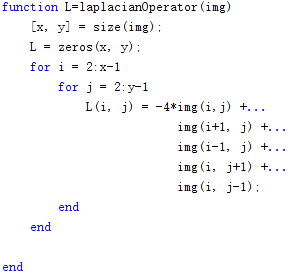voidwalker
 Note on paper "image inpainting - siggraph 2000"
• # Image inpainting

• ## ​METHOD

• ### ​express the information  $L$

• #### ​use discrete implementation of the Laplacian as estimator    $L^n(i,j) := I_{xx}^n(i,j)+I_{yy}^n(i,j)$使用拉普拉斯算子代表 像素 I(i,j)的信息/平滑程度。L(i,j)的信息等于它的上下左右四个像素之和减去该像素的四倍。

• ### ​express the direction  $N$

• #### ​use a time varying estimation of the isophotes direction field

% EXTRACTING ISOPHOTES FROM AN IMAGE
%
% Usage:  [ I, theta ] = isophote( L, alpha );
%
% Argument:   L     -  Luminance of the input image [0 255]
%             alpha -  Threshold of isophotes       [0 1]
%
% Returns:    I     -  Magnitude of the isophotes   [0 1]
%             theta -  Angle of the isophotes   [-pi/2 +pi/2]
% Vahid. K. Alilou
% Department of Computer Engineering
% The University of Semnan
%
% December 2014
function [ I, theta ] = isophote( L, alpha )
L = double(L)/255; theta=zeros(size(L));
I = sqrt(Lx.^2+Ly.^2);
I = I./max(max(I));
T = I>=alpha;
theta(T) = atan(Ly(T)./Lx(T));
I(I<alpha)=0;
end